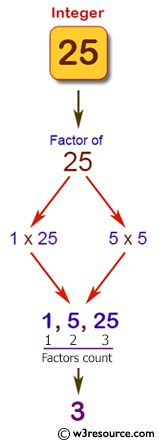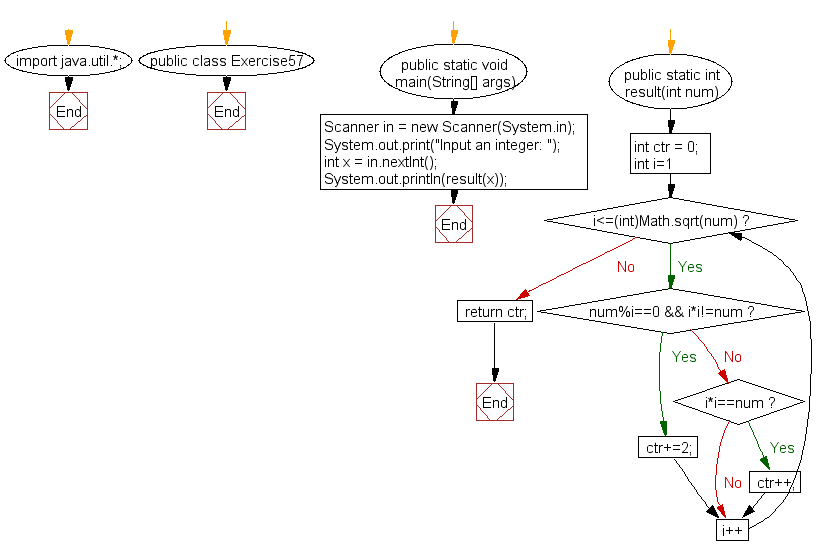﻿ Java: Accepts an integer and count the factors of the number# Java Exercises: Accepts an integer and count the factors of the number

## Java Basic: Exercise-57 with Solution

Write a Java program to accepts an integer and count the factors of the number.

Sample Solution:

Java Code:

``````import java.util.*;
public class Exercise57 {
public static void main(String[] args){
Scanner in = new Scanner(System.in);
System.out.print("Input an integer: ");
int x = in.nextInt();

System.out.println(result(x));
}
public static int result(int num) {
int ctr = 0;
for(int i=1; i<=(int)Math.sqrt(num); i++) {
if(num%i==0 && i*i!=num) {
ctr+=2;
} else if (i*i==num) {
ctr++;
}
}
return ctr;
}
}
```
```

Sample Output:

```Input an integer: 25
3
```

Pictorial Presentation:Flowchart:Java Code Editor:

What is the difficulty level of this exercise?

Test your Programming skills with w3resource's quiz.

﻿

## Java: Tips of the Day

countOccurrences

Counts the occurrences of a value in an array.

Use Arrays.stream().filter().count() to count total number of values that equals the specified value.

```public static long countOccurrences(int[] numbers, int value) {
return Arrays.stream(numbers)
.filter(number -> number == value)
.count();
}
```

Ref: https://bit.ly/3kCAgLb